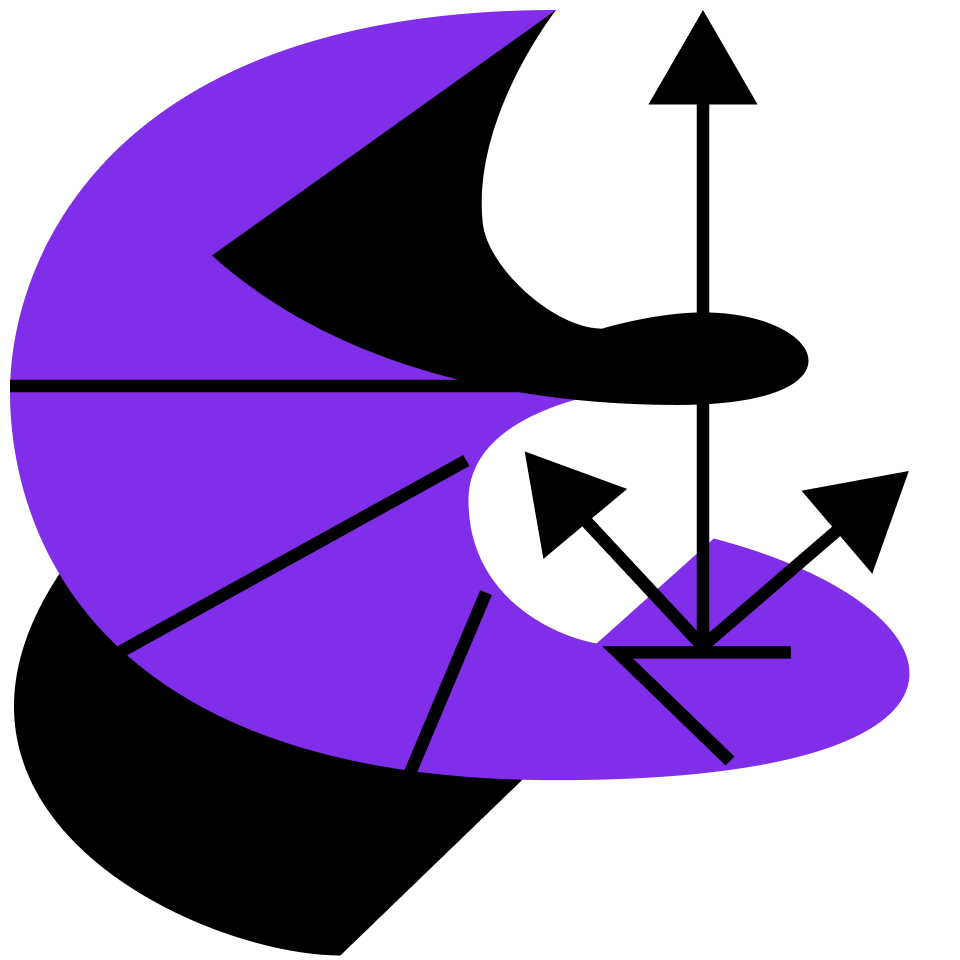## Vector Calculus

Extend multivariable calculus to vector fields, then apply your new skills by exploring Maxwell's equations.

Vector Calculus in a Nutshell

Calculus of Motion

Space Curves

Integrals and Arc Length

Frenet Formulae

Parametric Surfaces

Vector Fields

Jack and the Beanstalk

Electrostatic Bootcamp

Introducing Surface Integrals

Flux (Part I)

Flux (Part II)

Surface Integrals

Divergence (Part I)

Divergence (Part II)

The Divergence Theorem

The Divergence Theorem and Fluids

Flows & Divergence

Work (Part I)

Work (Part II)

Line Integrals

Path Independence

Curl

Stokes' Theorem

Swirls and Curls

Differential Forms (Optional)

The Laplacian

Gaussian Integrals (Part I)

Gaussian Integrals (Part II)

Fourier Transform

The Diffusion Equation

The Wave Equation

Maxwell's Equations (Part I)

Maxwell's Equations (Part II)

More Electrostatics!

### Course description

Change is deeply rooted in the natural world. Fluids, electromagnetic fields, the orbits of planets, the motion of molecules; all are described by vectors and all have characteristics depending on where we look and when. In this course, you'll learn how to quantify such change with calculus on vector fields. Go beyond the math to explore the underlying ideas scientists and engineers use every day.

### Topics covered

• Curl
• Differential Forms
• Divergence Theorem
• Flux
• Fourier Transform
• Line Integrals
• Maxwell's Equations
• Parametric Surfaces
• Space Curves
• Stokes' Theorem
• Surface Integrals
• Vector Fields

### Prerequisites and next steps

You’ll need a solid understanding of algebra, as well as the basics of functions, limits, derivatives, and integrals.

### Prerequisites

• Calculus Fundamentals
• Multivariable Calculus